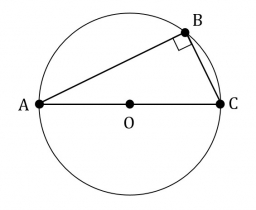# Right-angled 64084

A right-angled triangle ABC with sides 5 cm and 12 cm is described by circle k. Calculate the length of circle k in centimeters. When calculating, use π = 3, 14 and round the result to tenths.

o =  40.8 cm

### Step-by-step explanation:Did you find an error or inaccuracy? Feel free to write us. Thank you!

Tips for related online calculators